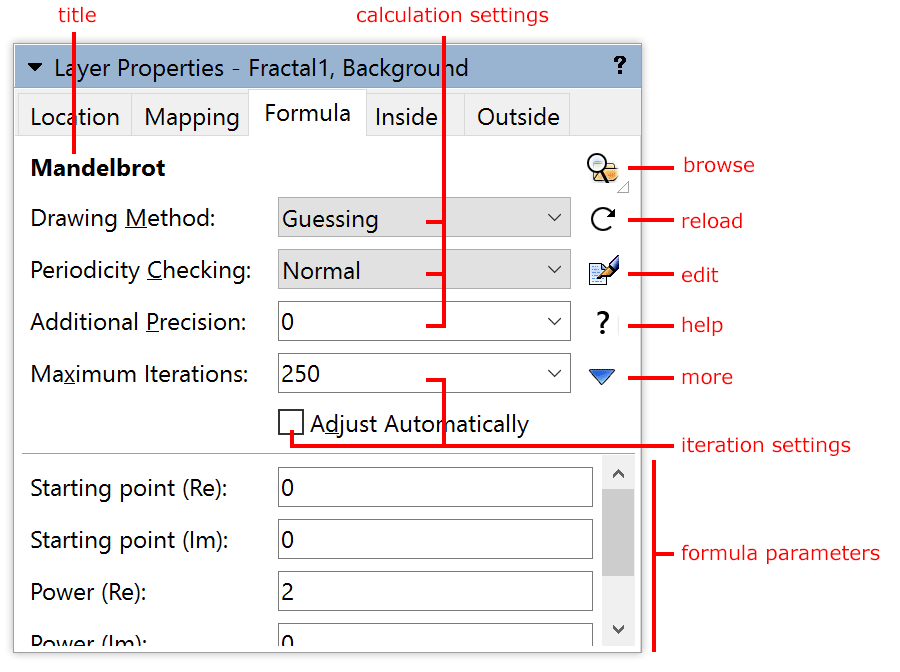# Fractal formulas

The fractal formula creates the basic shape and form of a fractal. Ultra Fractal comes with a number of standard formulas that you can use. You can also download additional formulas from the Internet and even write your own formulas.

Fractal formulas are managed in the Formula tab of the Layer Properties tool window.• At the top, the title of the fractal formula is shown. Hold the mouse cursor over the title to see the entry identifier and the file name of the formula.
• The Browse button opens a modal browser to select another fractal formula.
• The Reload button reloads the fractal formula from disk and recalculates the layer.
• The Edit button opens the fractal formula in the formula editor.
• The Help button opens the help file for the formula, if one exists.